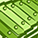# QlikView App Dev

Discussion Board for collaboration related to QlikView App Development.

Announcements
Join this live chat April 6, 10AM EST - QlikView to Qlik Sense REGISTER
cancel
Showing results for
Did you mean:Master

## why we use num here

Hi all,

I have a doubt like  in my script I have field

MonthName("Month") as           Test_Month,

num(MonthName("Month"))   as  Test_MonthNo,

in front end expression:

we are using like below.

Count({<Test_MonthNo= {\$(=max(Test_MonthNo))}>}ID)

so instead of month why we are using Test_monthno ?

is there any specific reason?  can anyone explain bit on this?

Thanks

Sony

1 Solution

Accepted SolutionsMVP

Yes, all of the above applies to something formatted with Date.  MonthName is a date as well.  It's equivalent to

Date(MonthStart(mydate), 'MMM YYYY')

Note that the MaxString() solution will only work for equals tests.  If you are testing less than or greater than you need to use the numeric form.

-Rob

http://qlikviewcookbook.com

10 RepliesContributor III

1.Test_Month gives you Jan, Feb, March

2. Test_MonthNo gives you the number of month corresponding to that month suppose Jan-1, Feb-2,.....

Regards,

AjayContributor III

Hi

Perhaps, Test_Month is a string, Test_MonthNo is a number.

So, max(Test_MonthNo) will get 12 (for example), max(Test_Month) will return 'September'.Master
Author

is that the only reason behind that or anything else?MVP

I think there is a small difference between these interpretations and reality.

MonthName(Date) returns the textname of the month/year of Date (according to the format set by the MonthName system variable), associated with the first millisecond of the first day of that month. That means that there is an invisible MonthStart() call executed by MonthName. For today that would be dual('Mar 2018', 43160)

Also Max() both expects and returns numerical values, with an added finesse that it only returns numerical values. The expression Text(Max(Test_Month)) will return the same value as Max(Test_Month) = 43160.

Set analysis doesn't want to select the maximum month of 12, but the maximum MonthYear value in your data set. Set analysis however is very picky on the format of the values you calculate and assign to fields. The formats must be identical. Therefore since you want to calculate the maximum MonthYear value, and you cannot compare this to a field that also contains a MonthName as text representation, you need a field with just the possible numerical values that may come out of the Max() call.MVP

I think you can also skip the Test_MonthNo field and use a set expression like

=Count({<Test_Month = {'\$(=MonthName(Max(Test_MonthNo)))'}>} ID)

but for now that's just a guess. I didn't test it (sorry Rob)MVP

You should have tested PeterAdding to what Peter has already said.

MonthName returns a Dual value with String of Month-Year and numeric of first day of the month.  Max() returns the numeric value.  Outside of a set expression, comparing a numeric to a dual is fine, QV will detect that you want to compare numeric.

=Test_Month = max(Test_Month)  // Returns True

Set expressions use search logic, it will only use the string portion of a dual.  So:

{<Test_Month= {\$(=max(Test_Month))}>}  // always false because 'Mar 2018' <> 43160

And that's why you are making an explicit numeric form of the date. So you can compare 43160 = 43160 in a set modifier.

-Rob

http://qlikviewcookbook.comMVP

Adding to the above replies, If you really want to use MonthName field then try

Count({<Test_Month= {"\$(=Maxstring(Test_Month))"}>}ID)Master
Author

the same way will work for Dates also?  like datenumMVP

Yes, all of the above applies to something formatted with Date.  MonthName is a date as well.  It's equivalent to

Date(MonthStart(mydate), 'MMM YYYY')

Note that the MaxString() solution will only work for equals tests.  If you are testing less than or greater than you need to use the numeric form.

-Rob